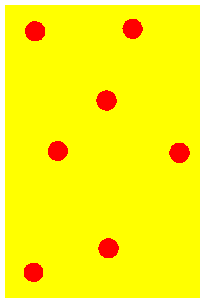# Kinetic Molecular Theory

16 Questions | Total Attempts: 887Settings.

• 1.
Each of the containers is shown at the same temperature. In which container is the average speed of the particles the fastest?
• A.

Hydrogen

• B.

Nitrogen

• C.

Oxygen

• D.

Chlorine

• 2.
The stoppers are removed from each container, which are in the same room at the same temperature. Which gas diffuses through the room the most slowly.
• A.

All at the same rate

• B.

Nitrogen

• C.

Hydrogen

• D.

Chlorine

• 3.
As the sealed, inflexible container is heated, the gas molecules inside..
• A.

Increase in volume

• B.

Begin to move faster

• C.

Condense to a liquide

• D.

Become less dense

• 4.
The stopper is removed from the container of chlorine, at which temperature will the gas diffuse the fastest.
• A.

290 K

• B.

273 K

• C.

245 K

• D.

310 K

• 5.
Which postulate of KMT explains why the volume (or pressure)  of a gas increases when you increase the temperature?
• A.

1‐ All particles are infinitely small and the size of a particle is negligible compared to the container that it holds.

• B.

2‐ The particles of gas are in constant motion and move in straight lines.

• C.

3- The particles of gas do not exert any force of attraction or repulsion on each other. There is no energy loss during a collision.

• D.

4‐ The mean kinetic energy of the particles is directly proportional to their absolute temperature.

• 6.
In a real gas particles have complete inelastic collisions meaning no energy is lost.
• A.

True

• B.

False

• 7.
Gases found in the environment are most likely to exhibit properties similar to that of ideal gases under conditions of:
• A.

High temperatures and high pressures

• B.

Low temperatures and low pressures

• C.

High temperatures and low pressures

• D.

Low temperatures and high pressures

• 8.
Which postulate of KMT explains why gases are so easily compressed?
• A.

1‐ All particles are infinitely small and the size of a particle is negligible compared to the container that it holds.

• B.

2- The particles of gas are in constant motion and move in straight lines.

• C.

3- The particles of gas do not exert any force of attraction or repulsion on each other. There is no energy loss during a collision.

• D.

4- The mean kinetic energy of the particles is directly proportional to their absolute temperature.

• 9.
According to the kinetic theory of gases, an ideal gas has all of the following characteristics but 1. Which one?
• A.

Particles whose size is nil or negligible

• B.

Particles that mutually attract one another

• C.

Particles that move randomly but in straight lines

• D.

Particles that are very far away from each other

• 10.
Which postulate of the KMT explains why gases do not have a fixed volume.
• A.

All particles are infinitely small and the size of a particle is negligible compared to the container that it holds.

• B.

The particles of gas are in constant motion and move in straight lines.

• C.

The particles of gas do not exert any force of attraction or repulsion on each other. There is no energy loss during a collision.

• D.

The mean kinetic energy of the particles is directly proportional to their absolute temperature.

• 11.
Which postulate of the KMT explains why the smell of skunk spray can seep into a house even when the windows are closed.
• A.

1‐ All particles are infinitely small and the size of a particle is negligible compared to the container that it holds.

• B.

2‐ The particles of gas are in constant motion and move in straight lines.

• C.

3‐ The particles of gas do not exert any force of attraction or repulsion on each other. There is no energy loss during a collision.

• D.

4‐ The mean kinetic energy of the particles is directly proportional to their absolute temperature.

• 12.
Which postulate of KMT explains why the scent of fresh coffee in the morning fills up every room in the house?
• A.

1-All particles are infinitely small and the size of a particle is negligible compared to the container that it holds.

• B.

2‐ The particles of gas are in constant motion and move in straight lines.

• C.

3‐ The particles of gas do not exert any force of attraction or repulsion on each other. There is no energy loss during a collision.

• D.

4‐ The mean kinetic energy of the particles is directly proportional to their absolute temperature.

• 13.
Which state of matter exhibits only vibrational movement?
• A.

Liquid

• B.

Solid

• C.

Gas

• D.

Plasma

• 14.
Particules will experience large velocities at ...
• A.

Low temperature

• B.

Intermediate temperature

• C.

High temperature

• D.

Option 4

• 15.
Which colour curve represents the lowest temperature
• A.

Blue

• B.

Purple

• C.

Red

• D.

Black

• 16.
What is the kinetic energy of a 35 g of oxygen traveling at a velocity of 650 m/s?
• A.

7,393,750 J

• B.

7,393,750 KJ

• C.

7393.75 J

• D.

7393.75 KJBack to top